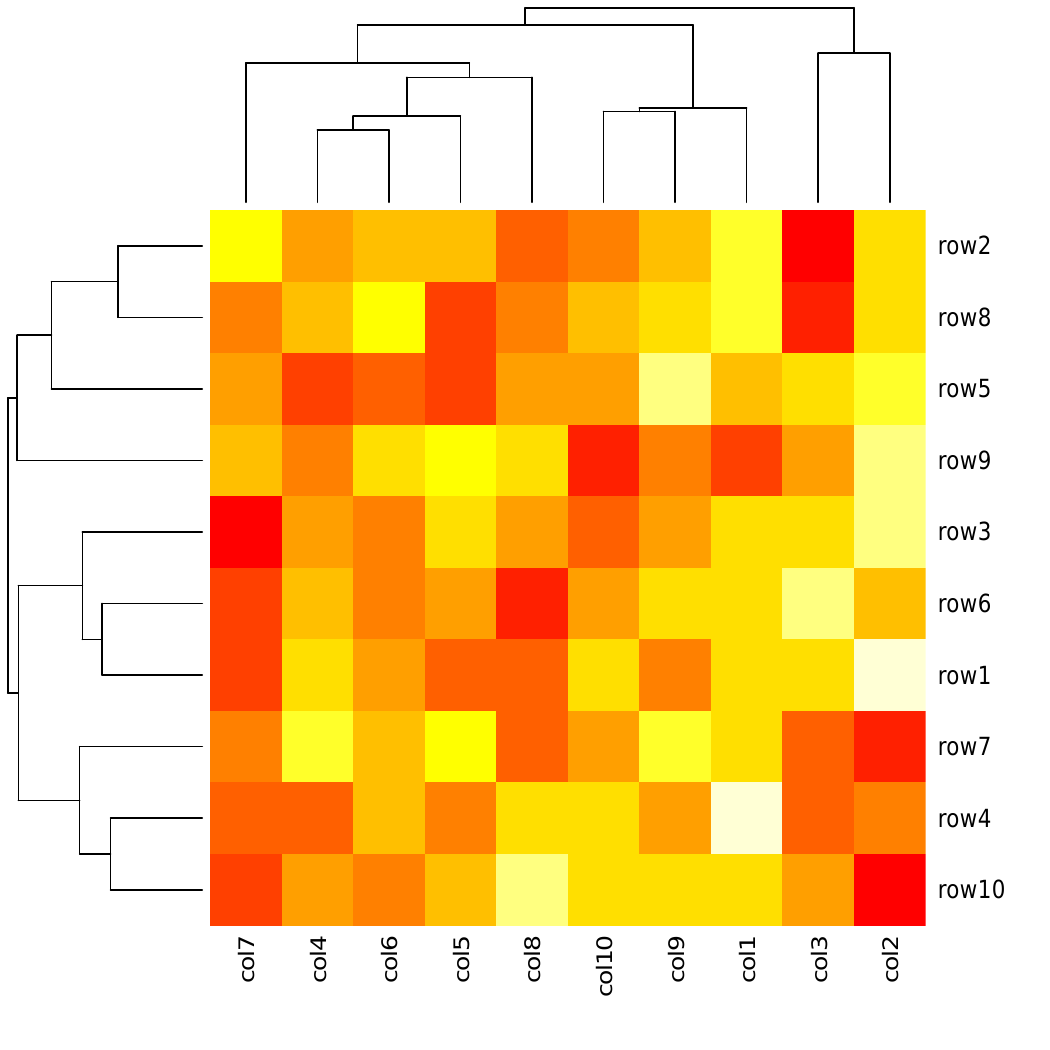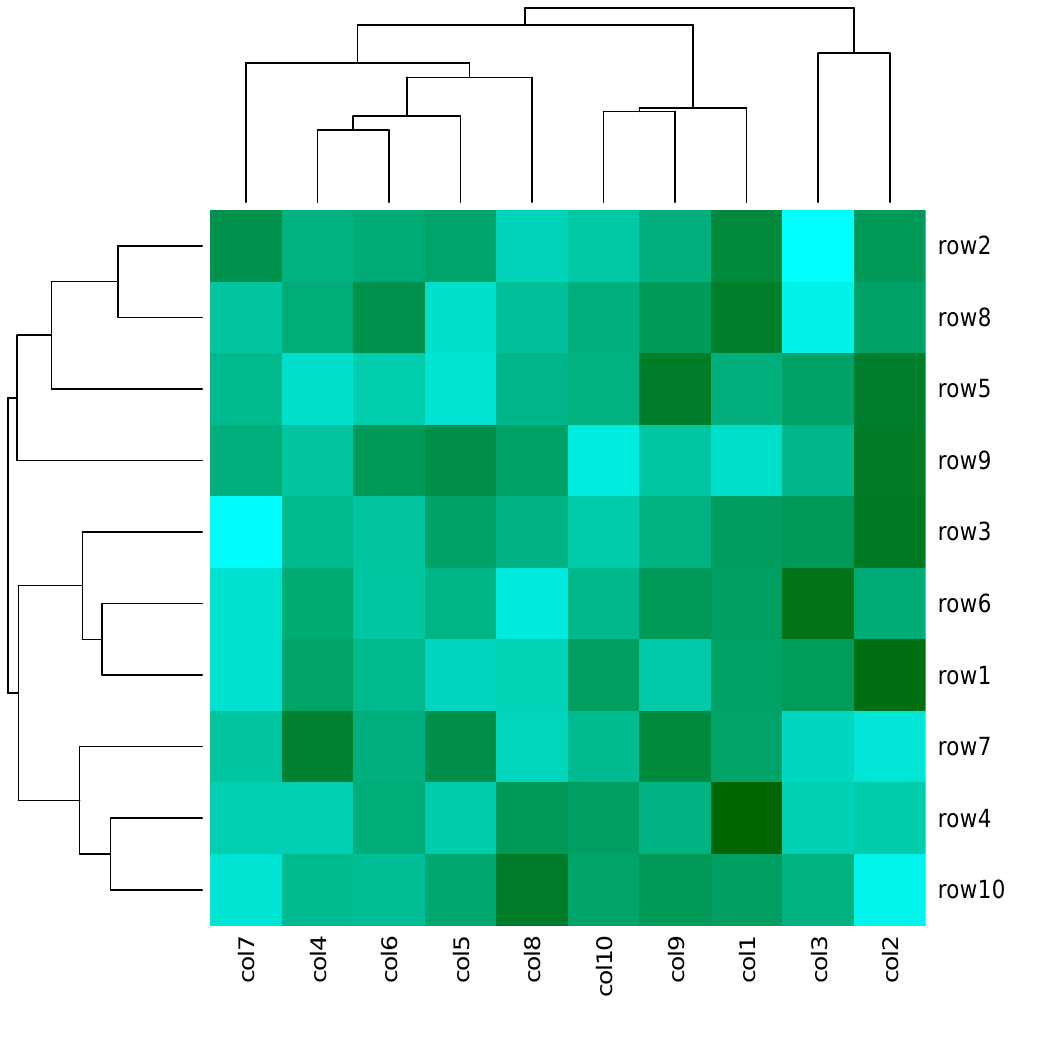# Create a Heatmap in R Programming – heatmap() Function

• Last Updated : 03 Jul, 2020

`heatmap()` function in R Language is used to plot a heatmap. Heatmap is defined as a graphical representation of data using colors to visualize the value of the matrix. In this to represent more common values or higher activities brighter colors basically reddish colors are used and to less common or activity values darker colors are preferred. Heatmap is also defined by the name of the shading matrix.

Syntax: heatmap(data)

Parameters:
data: It represent matrix data, such as values of rows and columns

Return: This function draws a heatmap.

Example 1:

 `# Set seed for reproducibility``set.seed``(110) `` ` `# Create example data``data <- ``matrix``(``rnorm``(100, 0, 5), nrow = 10, ncol = 10) `` ` `# Column names``colnames``(data) <- ``paste0``(``"col"``, 1:10)``rownames``(data) <- ``paste0``(``"row"``, 1:10)   `` ` `# Draw a heatmap``heatmap``(data)        `

Output:Here, in above example number of rows and columns are specified to draw heatmap with a given function.

Example 2:

 `# Set seed for reproducibility``set.seed``(110)   `` ` `# Create example data        ``data <- ``matrix``(``rnorm``(100, 0, 5), nrow = 10, ncol = 10)   `` ` `# Column names       ``colnames``(data) <- ``paste0``(``"col"``, 1:10)``rownames``(data) <- ``paste0``(``"row"``, 1:10)   `` ` `# Remove dendogram``# Manual color range``my_colors <- ``colorRampPalette``(``c``(``"cyan"``, ``"darkgreen"``)) `` ` `# Heatmap with manual colors``heatmap``(data, col = ``my_colors``(100))                             `

Output:In the above example heat map is drawn by using colorRampPalette to merge two different colors.

My Personal Notes arrow_drop_up